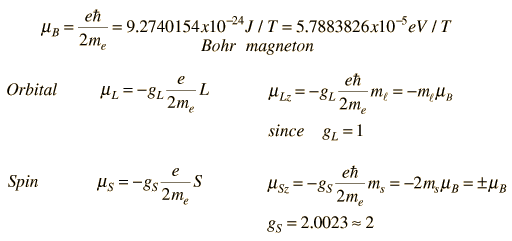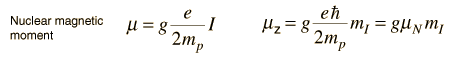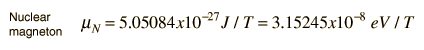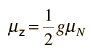# Nuclear Spin

It is common practice to represent the total angular momentum of a nucleus by the symbol I and to call it "nuclear spin". For electrons in atoms we make a clear distinction between electron spin and electron orbital angular momentum, and then combine them to give the total angular momentum. But nuclei often act as if they are a single entity with intrinsic angular momentum I. Associated with each nuclear spin is a nuclear magnetic moment which produces magnetic interactions with its environment.

The nuclear spins for individual protons and neutrons parallels the treatment of electron spin, with spin 1/2 and an associated magnetic moment. The magnetic moment is much smaller than that of the electron. For the combination neutrons and protons into nuclei, the situation is more complicated.

A characteristic of the collection of protons and neutrons (which are fermions) is that a nucleus of odd mass number A will have a half-integer spin and a nucleus of even A will have integer spin. The suggestion that the angular momenta of nucleons tend to form pairs is supported by the fact that all nuclei with even Z and even N have nuclear spin I=0. For example, in the nuclear data table for iron below, all the even A nuclides have spin I=0 since there are even numbers of both neutrons and protons. The half-integer spins of the odd-A nuclides suggests that this is the nuclear spin contributed by the odd neutron.

Isotopes of Iron
 Z A AtomicMass (u) NuclearMass(GeV/c2 Binding Energy(MeV) Spin NaturalAbund. Half-life Decay QMeV 26 54 53.939613 50.2315 471.77 0 0.059 stable ... ... 26 55 54.938296 51.1618 481.07 3/2 ... 2.7y EC 0.23 26 56 55.934939 52.0902 492.26 0 0.9172 stable ... ... 26 57 56.935396 53.0221 499.91 1/2 0.021 stable ... ... 26 58 57.933277 53.9517 509.96 0 0.0028 stable ... ... 26 60 59.934077 55.8154 525.35 0 ... 1.5My b- 0.24

The nuclear data of cobalt, just above iron in the periodic table, shows dramatically different nuclear spins I. The nuclides with even neutron number show half-integer spins associated with the odd proton, while those with odd neutron number show large integer spins associated with the two nucleons which are unpaired.

Isotopes of Cobalt
 Z A AtomicMass (u) NuclearMass(GeV/c2 Binding Energy(MeV) Spin NaturalAbund. Half-life Decay QMeV 27 56 55.939841 52.0943 486.92 4 ... 77.7d b+ 4.57 27 57 56.936294 53.0225 498.29 7/2 ... 271d EC 0.84 27 59 58.933198 54.8826 517.32 7/2 1.00 stable ... ... 27 60 59.933820 55.8147 524.81 5 ... 5.272y b- 2.82
 Nuclear magnetic moments
Index

Nuclear Structure Concepts

Reference
Krane
Ch 3

 HyperPhysics***** Nuclear R Nave
Go Back

# Nuclear Magnetic Moments

Associated with each nuclear spin is a magnetic moment which is associated with the angular momentum of the nucleus. It is common practice to express these magnetic moments in terms of the nuclear spin in a manner parallel to the treatment of the magnetic moments of electron spin and electron orbital angular momentum.

For the electron spin and orbital cases, the magnetic moments are expressed in terms of a unit called a Bohr magneton which arises naturally in the treatment of quantized angular momentum.Generally, the measured quantity is proportional to the z-component of the magnetic moment (the component along the experimentally determined direction such as the direction of an applied magnetic field, etc. ). In this treatment, the use of a "gyromagnetic ratio" or "g-factor" is introduced. The g-factor for orbital is just gL = 1, but the electron spin g-factor is approximately gS = 2.

For the nuclear case we proceed in a parallel manner. The nuclear magnetic moment is expressed in terms of the nuclear spin in the formwhere we have now introduced a new unit called a nuclear magneton.For free protons and neutrons with spin I =1/2, the magnetic moments are of the formwhere

Proton: g = 5.5856947

In 2014 a direct measurement of the magnetic moment of the proton gave 2.792847350(9) nuclear magnetons. (Mooser et al, Nature, May 29, 2014). Efforts are underway to measure the magnetic moment of the antiproton with comparable accuracy, since a measured difference between the proton and antiproton could be a valuable clue toward unraveling the mystery of why matter greatly dominates antimatter in the universe (antimatter problem).

Neutron: g = -3.8260837 +/- 0.0000018

The proton g-factor is far from the gS = 2 for the electron, and even the uncharged neutron has a sizable magnetic moment! For the neutron, this suggests that there is internal structure involving the movement of charged particles, even though the net charge of the neutron is zero. If g=2 were an expected value for the proton and g=0 were expected for the neutron, then it was noted by early researchers that the the proton g-factor is 3.6 units above its expected value and the neutron value is 3.8 units below its expected value. This approximate symmetry was used in trial models of the magnetic moment, and in retrospect is taken as an indication of the internal structure of quarks in the standard model of the proton and neutron.

Note that the maximum effective magnetic moment of a nucleus in nuclear magnetons will be the g-factor multiplied by the nuclear spin. For a proton with g = 5.5857 the quoted magnetic moment is m = 2.7928 nuclear magnetons.

 Nuclide Nuclear spin I Magnetic momentm in mN n 1/2 -1.9130418 p 1/2 +2.7928456 2H(D) 1 +0.8574376 17O 5/2 -1.89279 57Fe 1/2 +0.09062293 57Co 7/2 +4.733 93Nb 9/2 +6.1705

Data from V. S. Shirley, Table of Isotopes, Wiley, New York, 1978, Appendix VII.

 Nuclear magnetic resonance
Index

Nuclear Structure Concepts

Reference
Krane
Ch 3

 HyperPhysics***** Nuclear R Nave
Go Back